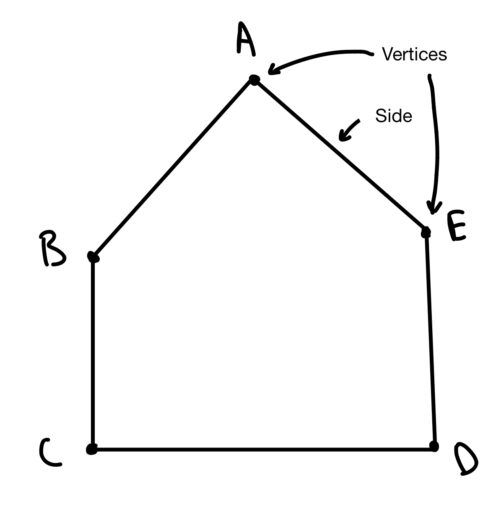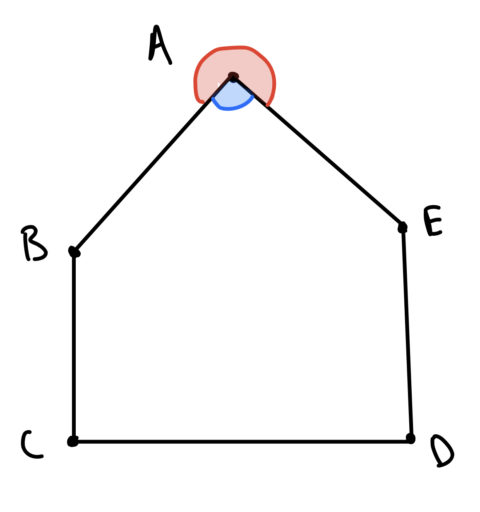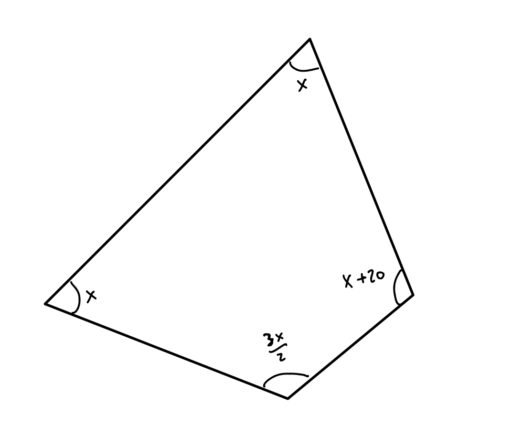Now, with respect to triangles, there are two main facts you need to know:

Triangles have 180 degrees: The angles of a triangle add up to 180 degrees.

Reverse Pythagorean Theorem: Triangles whose side lengths obey the Pythagorean Theorem (i.e. the triangle has side lengthsand) must have a right angle.

Sometimes, a problem will be somewhat sneaky: instead of directly telling you that a triangle is a right triangle, it will give you the side lengths and leave you to make that inference. (Recall here our Reverse Pythagorean Theorem)

The final fact which can help in finding an unknown angle is the following formula for the sum of all the angles of a polygon:

Polygon Angle Sum Formula: For an-sided 2D shape, the sum of all the interior angles is equal toWhat is an interior angle? Well on a polygon, we have some sides and some vertices (points where the sides meet). For example:At each vertex, there is are two angles:The interior angle, sensibly enough, is the angle that is insidethe polygon. In the above diagram, it is the blue angle.

Thus, our formula lets us calculate, for any polygon, the sum of all of its interior angles. This can be helpful when you are given almost all of the angles of a polygon and you need to find the final angle.

Practice Problems

1. What is the sum of the interior angles for a 13-sided polygon?

2. Find the value of any interior angle of a regular 14-sided polygon.

3. Find the angles of the below polygon: